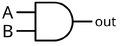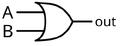## FANDOM

68 Pages

A logic gate performs a logical operation on one or more logic inputs and produces a single logic output. The logic normally performed is Boolean logic and is most commonly found in digital circuits. Logic gates are primarily implemented electronically using diodes or transsitors, but can also be constructed using electromagnetic relays, fluidics, optics, or even mechanical elements.

## Logic gatesEdit

A logic gate takes one or more logic-level inputs and produces a single logic-level output. Because the output is also a logic level, an output of one logic gate can connect to the input of one or more other logic gates. Two outputs are never connected together as they could produce conflicting logic values. In electronic logic gates, this would cause a short circuit.

In electronic logic, a logic level is represented by a certain voltage ( this is wrong . Each logic gate requires power so that it can source and sink currents to achieve the correct output voltage. In logic circuit diagrams the power is not shown, but in a full electronic schematic, power connections are required.

The simplest form of electronic logic is logic. This allows AND and OR gates to be built, but not inverters, and so is an incomplete form of logic. To build a complete logic system, a or can be used. The simplest family of logic gates using bipolar transistors is called meta data-rte-instance, from a change in input of a gate to the corresponding change in its output. When gates are cascaded, the total propagation delay is approximately the sum of the individual delays, an effect which can become a problem in high-speed circuits.

## Logic gates and hardwareEdit

NAND and NOR logic gates are the two pillars of logic, in that all other types of Boolean logic gates (i.e., AND, OR, NOT, XOR, XNOR) can be created from a suitable network of just NAND or just NOR gate(s). They can be built from relays or transistors, or any other technology that can create an inverter and a two-input AND or OR gate. Hence the NAND and NOR gates are called the universal gates.

For an input of 2 variables, there are 16 possible boolean algebraic functions. These 16 functions are enumerated below, together with their outputs for each combination of inputs variables.

 INPUT A 0 0 1 1 B 0 1 0 1 OUTPUT 0 0 0 0 0 A AND B 0 0 0 1 A > B * 0 0 1 0 A 0 0 1 1 A < B * 0 1 0 0 B 0 1 0 1 A XOR B 0 1 1 0 A OR B 0 1 1 1 A NOR B 1 0 0 0 A XNOR B 1 0 0 1 NOT B 1 0 1 0 A ≥ B * 1 0 1 1 NOT A 1 1 0 0 A ≤ B * 1 1 0 1 A NAND B 1 1 1 0 1 1 1 1 1

The four functions labeled with a "*" are the logical implication functions: "A ≤ B" can be read as "A implies B"; it follows that "A > B" is "A does not imply B". These four functions are less common and are usually not implemented directly as logic gates.

Logic gates are a vital part of many digital circuits, and as such, every kind is available as an IC. For examples, see the 4000 series of CMOS logic chips or the 700 series.

## SymbolsEdit

There are two sets of symbols in common use, both now defined by ANSI/IEEE Std 91-1984 and its supplement ANSI/IEEE Std 91a-1991. The "distinctive shape" set, based on traditional schematics, is used for simple drawings and is quicker to draw by hand. It is sometimes unofficially described as "military", reflecting its origin if not its modern usage. The "rectangular shape" set, based on IEC 60617-12, has rectangular outlines for all types of gate, and allows representation of a much wider range of devices than is possible with the traditional symbols. The IEC's system has been adopted by other standards, such as EN 60617-12:1999 in Europe and BS EN 60617-12:1999 in the United Kingdom.

Type Distinctive shape Rectangular shape Boolean algebra between A & B Truth table
AND$A \cdot B$
 INPUT OUTPUT A B A AND B 0 0 0 0 1 0 1 0 0 1 1 1
OR$A+B$
 INPUT OUTPUT A B A OR B 0 0 0 0 1 1 1 0 1 1 1 1
NOT$\overline{A}$
 INPUT OUTPUT A NOT A 0 1 1 0
In electronics a NOT gate is more commonly called an inverter. The circle on the symbol is called a bubble, and is generally used in circuit diagrams to indicate an inverted input or output.
NAND$\overline{A \cdot B}$
 INPUT OUTPUT A B A NAND B 0 0 1 0 1 1 1 0 1 1 1 0
NOR$\overline{A + B}$
 INPUT OUTPUT A B A NOR B 0 0 1 0 1 0 1 0 0 1 1 0
In practice, the cheapest gate to manufacture is usually the NAND gate. Additionally, Charles Peirce showed that NAND gates alone (as well as NOR gates alone) can be used to reproduce all the other logic gates.

Symbolically, a NAND gate can also be shown using the OR shape with bubbles on its inputs, and a NOR gate can be shown as an AND gate with bubbles on its inputs. This reflects the equivalency due to De Morgans law, but it also allows a diagram to be read more easily, or a circuit to be mapped onto available physical gates in packages easily, since any circuit node that has bubbles at both ends can be replaced by a simple bubble-less connection and a suitable change of gate. If the NAND is drawn as OR with input bubbles, and a NOR as AND with input bubbles, this gate substitution occurs automatically in the diagram (effectively, bubbles "cancel"). This is commonly seen in real logic diagrams - thus the reader must not get into the habit of associating the shapes exclusively as OR or AND shapes, but also take into account the bubbles at both inputs and outputs in order to determine the "true" logic function indicated.

Two more gates are the exclusive-OR or XOR function and its inverse, exclusive-NOR or XNOR. The two input Exclusive-OR is true only when the two input values are different, false if they are equal, regardless of the value. If there are more than two inputs, the gate generates a true at its output if the number of trues at its input is odd (). In practice, these gates are built from combinations of simpler logic gates.

XOR$A \oplus B$
 INPUT OUTPUT A B A XOR B 0 0 0 0 1 1 1 0 1 1 1 0
XNOR$\overline{A \oplus B}$
 INPUT OUTPUT A B A XNOR B 0 0 1 0 1 0 1 0 0 1 1 1

### List of Logic Gate ICsEdit

The 7400 series has several ICs that contain just four-input logic gates:

IC No. Gate
7400 NAND
7402 NOR
7404 NOT
7408 AND
7432 OR
7486 EX-OR

## DeMorgan equivalent symbolsEdit

By use of De Morgan's theorem, an AND gate can be turned into an OR gate by inverting the sense of the logic at its inputs and outputs. This leads to a separate set of symbols with inverted inputs and the opposite core symbol. These symbols can make circuit diagrams for circuits using active low signals much clearer and help to show accidental connection of an active high output to an active low input or vice-versa.

## Storage of bits 8 in a byteEdit

Related to the concept of logic gates (and also built from them) is the idea of storing a bit of information. The gates discussed up to here cannot store a value: when the inputs change, the outputs immediately react. It is possible to make a storage element either through a capacitor (which stores charge due to its physical properties) or by feedback. Connecting the output of a gate to the input causes it to be put through the logic again, and choosing the feedback correctly allows it to be preserved or modified through the use of other inputs. A set of gates arranged in this fashion is known as a "latch", and more complicated designs that utilise clocks (signals that oscillate with a known period) and change only on the rising edge are called edge-triggered "flip-flops". The combination of multiple flip-flops in parallel, to store a multiple-bit value, is known as a register. When using any of these gate setups the overall system has memory; it is then called a sequential system since its output can be influenced by its previous state(s).

These registers or capacitor-based circuits are known as computer memory. They vary in performance, based on factors of speed, complexity, and reliability of storage, and many different types of designs are used based on the application.

## Three-state logic gatesEdit

Three-state, or 3-state, logic gates have three states of the output: high (H), low (L) and high-impedance (Z). The high-impedance state plays no role in the logic, which remains strictly binary. These devices are used on buses to allow multiple chips to send data. A group of three-states driving a line with a suitable control circuit is basically equivalent to a multiplexer, which may be physically distributed over separate devices or plug-in cards.

'Tri-state', a widely-used synonym of 'three-state', is a trademark of the National Semiconductor Corporation.

## MiscellaneousEdit

Logic circuits include such devices as multiplexers, registers, arithmetic logic unitarithmetic units (ALUs), and computer memory, all the way up through complete microprocessors which can contain more than a 100 million gates. In practice, the gates are made from field effect transistors (FETs), particularly metal-oxide-semiconductor FETs (MOSFETs).

In reversible logic, Toffoli gates are used.

## History and developmentEdit

The earliest logic gates were made mechanically. Charles Babbage, around 1837, devised the Analytical Engine. His logic gates relied on mechanical gearing to perform operations. Electromagnetic relays were later used for logic gates. In 1891, Almon Strowger patented a device containing a logic gate switch circuit (Template:US patent). Strowger's patent was not in widespread use until the 1920s. Starting in 1898, Nikola Tesla filed for patents of devices containing logic gate circuits (see List of Tesla patents). Eventually, vacuum tubes replaced relays for logic operations. Lee De Forest's modification, in 1907, of the Fleming valve can be used as AND logic gate. Ludwig Wittgenstein introduced a version of the 16-row truth table in #Logic gates and hardware above, as proposition 5.101 of Tractatus Logico-Philosophicus (1921). Claude E. Shannon introduced the use of Boolean algebra in the analysis and design of switching circuits in 1937. Walther Bothe, inventor of the coincidence circuit, got part of the 1954 Nobel Prize in physics, for the first modern electronic AND gate in 1924. Active research is taking place in molecular logic gates.

##Edit

Community content is available under CC-BY-SA unless otherwise noted.